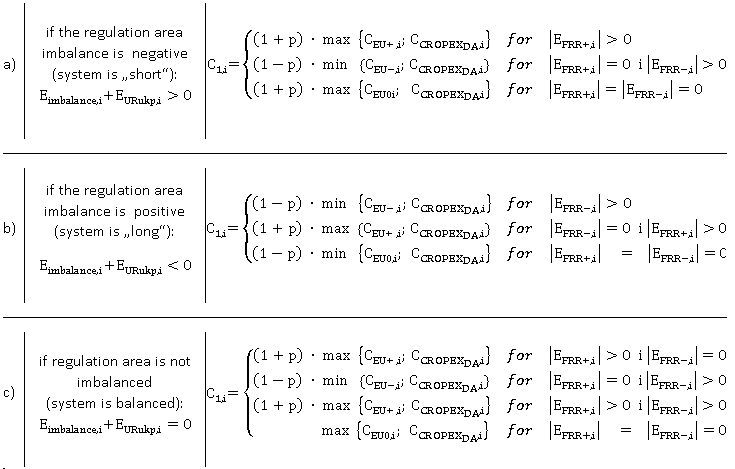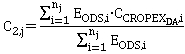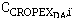## Financial clearing

The imbalance price in the first (monthly) imbalance settlement

The imbalance settlement is calculated with the imbalance price (C1,i) which is equal for all balance group representatives at settlement interval „i“ no matter if they have positive or negative imbalances. All prices and financial amounts are rounded to two decimal places.
The imbalance price at the settlement interval „i“ depends on the regulation area imbalance direction.. The regulation area imbalance is  the sum of the system imbalances (Eimbalance,i) and the total activated balancing energy in the regulation area (EURukp,i).
For the settlement interval „i“ the imbalance price C1,i) is equal:Where:
p                       –       is the coefficient of the financial neutrality (number between 0 and 1)
CEU+,i                –       is the weighted average price of the positive balancing energy activated from energy reserves for the purpose of frequency re-establishment on the transmission system, at the settlement interval [HRK/MWh]
CEU–,i                        –       is the weighted average price of the negative balancing energy activated from energy reserves for the purpose of frequency re-establishment on the transmission system, at the settlement interval [HRK/MWh]
–       is the day-ahead price of electricity on the Croatian Power Exchange (http://www.cropex.hr) at settlement interval „i“ [HRK/MWh]
EFRR+,i             –       is the total quantity of the realized positive balancing energy activated from power reserves at settlement interval „i“ [MWh]
EFRR,i             –       is the total quantity of the realized negative balancing energy activated from power reserves at settlement interval „i“ [MWh]
The coefficient of the financial neutrality is determined by the iterative process in steps, increasing „p“ by 0,1, starting from 0 till 1. The iterative process stops when the sum of all financial obligations made by all balance group representatives is lesser or equal to financial obligations of the transmission system operator for the activated balancing energy in the settlement period (month). The coefficient of the financial neutrality at the settlement interval “i” is equal to 0 (zero) if the weighted average price of the positive and/or negative balancing energy is negative.

The imbalance prices in the second (annual) imbalance settlement

The imbalance settlement charge is calculated with the imbalance price (C2,j) which is equal for both positive and negative imbalances in the settlement period „j“ and it is calculated in accordance with the following formula:Where:

EODS,i              –       is the value of the load curve of the distribution system determined by the Rules of application of replacement curves, at the settlement interval „i“ [MWh]–     is the day-ahead price of electricity on the Croatian Power Exchange (http://www.cropex.hr), at the settlement interval „i“ [HRK/MWh]
nj                      –       is the number of total settlement intervals for the settlement period (month) „j“ in which the day ahead price of electricity ( ) is available.

Invoicing for the first (monthly) and the second(annual) imbalance settlement

The transmission system operator invoices the balance group representative if the total financial obligation for the balance group imbalances is negative.

The balance group representative invoices the transmission system operator if the total financial obligation for the balance group imbalances is positive.